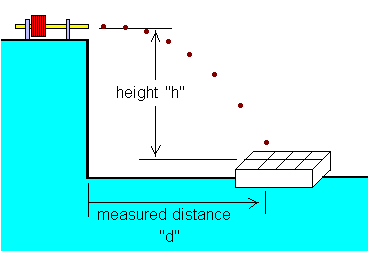# Horizontal Ballistic Speed Trap

If you fire the coilgun horizontally off a table, and measure the distance to where it lands, and the height it fell, then you have enough information to calculate the speed. Why? Because if we neglect air friction then the projectile follows a perfect parabolic arc. There is only one possible starting speed that can reach that distance from that height under standard gravity. We can solve the equations of motion to find the speed.Measure the height down to where it lands. This picture shows how the measurements are taken.

Speed = d * SQRT(g / 2h)
where d is horizontal distance in feet (or meters)
and h is vertical distance in feet (or meters)
and SQRT is the square root function

You can use English or metric units. However, your value of gravity must use the same units as your measurements.

• English: g = 32 feet per second2
• Metric:  g = 9.8 meters per second2

I prepared a cardboard box with dividers, by removing the beer bottles and "disposing" of their contents. No sacrifice is too great in the name of science.

 < Previous Page 2 of 9 Next >